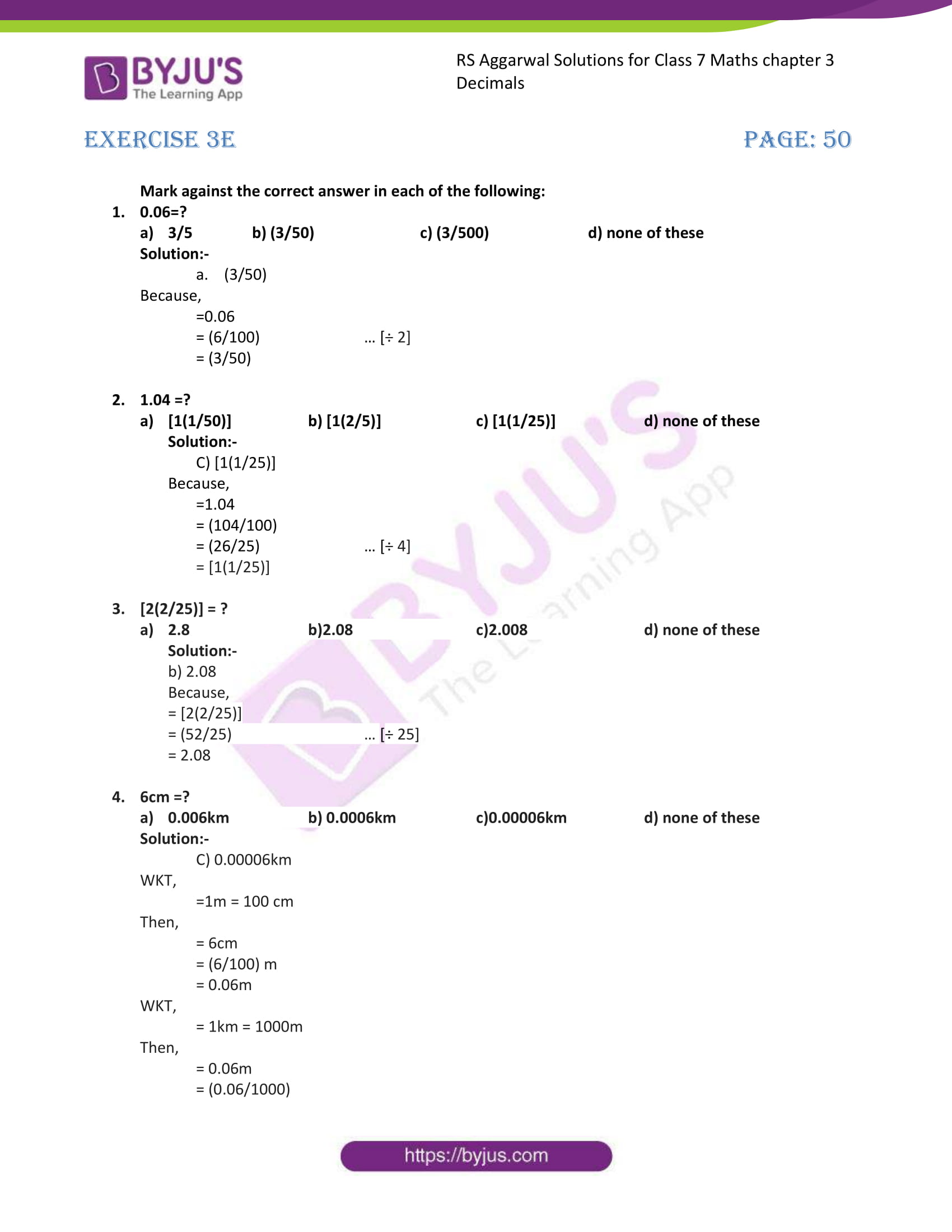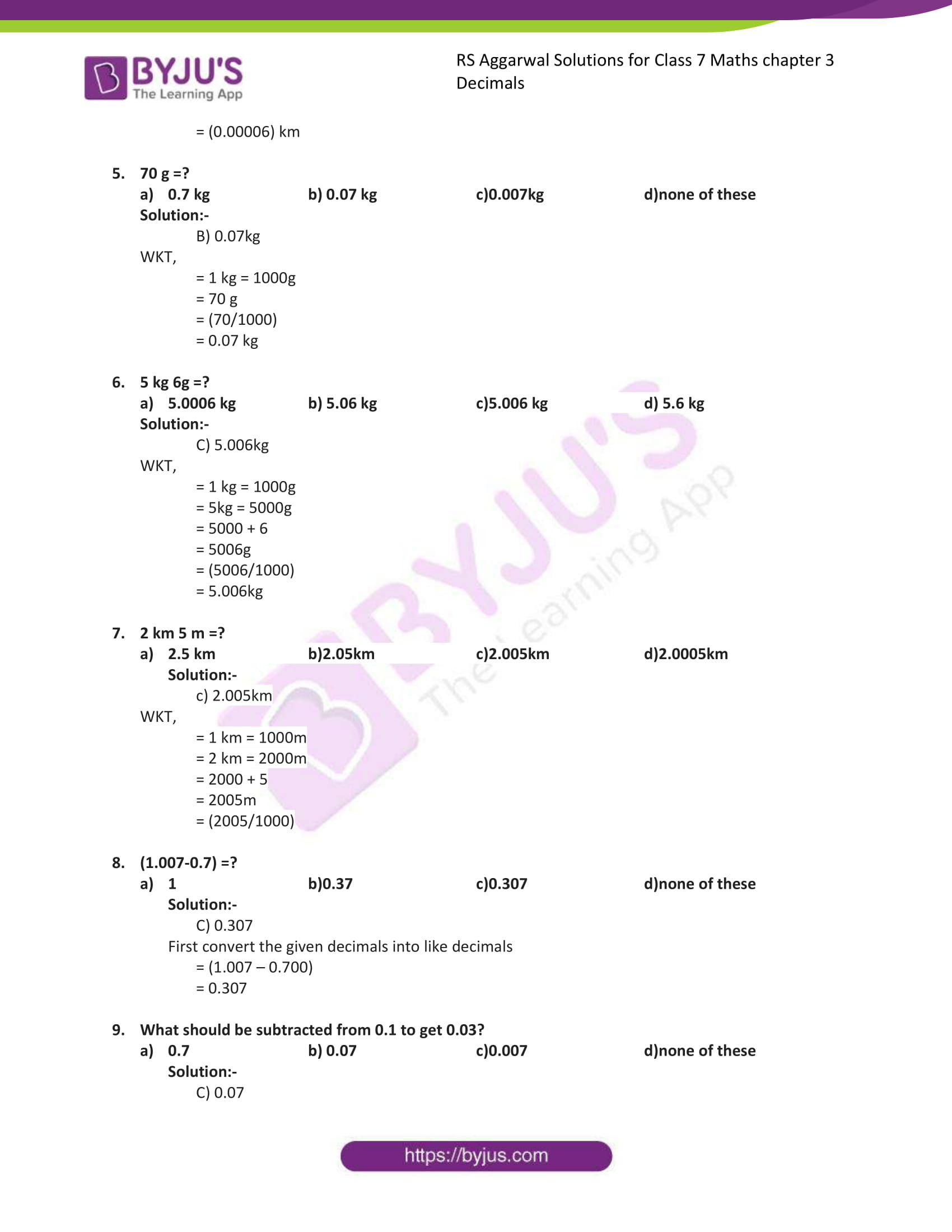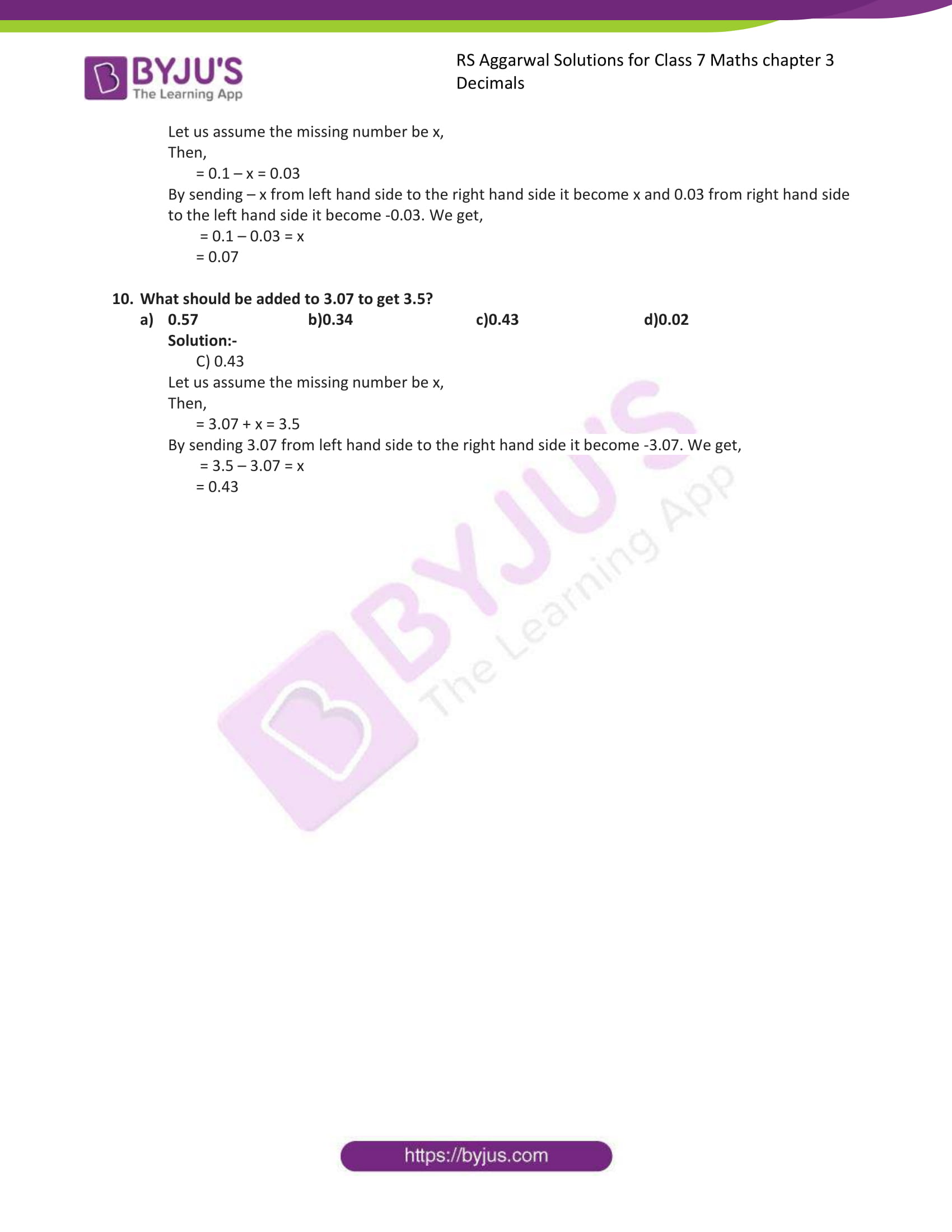# RS Aggarwal Solutions for Class 7 Maths Exercise 3E Chapter 3 Decimals

RS Aggarwal Solutions for Class 7 Maths Exercise 3E Chapter 3 Decimals in simple PDF are available here. This exercise of RS Aggarwal Solutions for Class 7 Chapter 3 Decimals contains objective type questions, which covers all the topics in the chapter with different types of questions. Students who go through these questions can solve any type of questions. Students are suggested to try solving the questions from RS Aggarwal Solutions for Class 7 Maths Exercise 3E Chapter 3 Decimals.

## Download the PDF of RS Aggarwal Solutions For Class 7 Maths Chapter 3 Decimals – Exercise 3E### Access answers to Maths RS Aggarwal Solutions for Class 7 Chapter 3 – Decimals Exercise 3E

1. 0.06=?

a)3/5 b)(3/50) c)(3/500) d)none of these

Solution:-

a. (3/50)

Because,

=0.06

= (6/100) … [÷ 2]

= (3/50)

2. 1.04 =?

a) [1(1/50)] b)[1(2/5)] c)[1(1/25)] d)none of these

Solution:-

C) [1(1/25)]

Because,

=1.04

= (104/100)

= (26/25) … [÷ 4]

= [1(1/25)]

3. [2(2/25)] = ?

a)2.8 b)2.08 c)2.008 d)none of these

Solution:-

b) 2.08

Because,

= [2(2/25)]

= (52/25) … [÷ 25]

= 2.08

4. 6cm =?

a)0.006km b)0.0006km c)0.00006km d)none of these

Solution:-

C) 0.00006km

WKT,

=1m = 100 cm

Then,

= 6cm

= (6/100) m

= 0.06m

WKT,

= 1km = 1000m

Then,

= 0.06m

= (0.06/1000)

= (0.00006) km

5. 70 g =?

a)0.7kg b)0.07kg c)0.007kg d)none of these

Solution:-

B) 0.07kg

WKT,

= 1 kg = 1000g

= 70 g

= (70/1000)

= 0.07 kg

6. 5kg 6g =?

a)5.0006kg b) 5.06kg c)5.006kg d) 5.6kg

Solution:-

C) 5.006kg

WKT,

= 1 kg = 1000g

= 5kg = 5000g

= 5000 + 6

= 5006g

= (5006/1000)

= 5.006kg

7. 2 km 5 m =?

a)2.5km b)2.05km c)2.005km d)2.0005km

Solution:-

c) 2.005km

WKT,

= 1 km = 1000m

= 2 km = 2000m

= 2000 + 5

= 2005m

= (2005/1000)

8. (1.007-0.7) =?

a)1 b)0.37 c)0.307 d)none of these

Solution:-

C) 0.307

First convert the given decimals into like decimals

= (1.007 – 0.700)

= 0.307

9. What should be subtracted from 0.1 to get 0.03?

a)0.7 b) 0.07 c)0.007 d)none of these

Solution:-

C) 0.07

Let us assume the missing number be x,

Then,

= 0.1 – x = 0.03

By sending – x from left hand side to the right hand side it become x and 0.03 from right hand side to the left hand side it become -0.03. We get,

= 0.1 – 0.03 = x

= 0.07

10. What should be added to 3.07 to get 3.5?

0.57 b)0.34 c)0.43 d)0.02

Solution:-

C) 0.43

Let us assume the missing number be x,

Then,

= 3.07 + x = 3.5

By sending 3.07 from left hand side to the right hand side it become -3.07. We get,

= 3.5 – 3.07 = x

= 0.43

### Access other exercises of RS Aggarwal Solutions For Class 7 Chapter 3 – Decimals

Exercise 3D Solutions